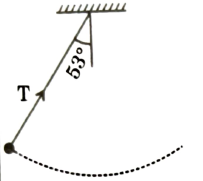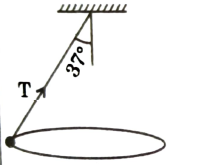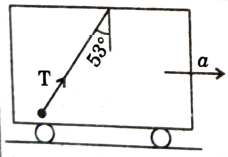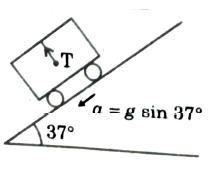Inertial and uniformly accelerated frame of reference
Question

# Match the column-I to value of Tension in column-Il assuming mass of particle equal to mColumn-IColumn-II(a)Extreme position in simple pendulum(p)$\frac{4\mathrm{mg}}{5}$(b)Conical pendulum(q)$\frac{5\mathrm{mg}}{4}$(c)Cart is accelerated such that particle remains at rest as shown w.r.t. cart.(r)$\frac{3\mathrm{mg}}{5}$(d)Cart is accelerating freely on the incline. Particle remains at rest w.r.t. cart as shown.(s)$\frac{5\mathrm{mg}}{3}$

Moderate
Solution

## (a) As velocity = 0    $⇒$No radial acceleration(b) Motion in horizontal plane   $⇒$no acceleration in vertical direction(c) Acceleration of particle w.r.t. cart is zero(d)  $\mathrm{T}=\mathrm{mgcos}{37}^{\circ }$

Get Instant Solutions